# The GLMPOWER Procedure

### CONTRAST Statement

• CONTRAST ’label’ effect values <…effect values> </ options>;

The CONTRAST statement enables you to define custom Type III hypothesis tests by specifying an
vector or matrix for testing either the hypothesis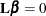(for univariate models) or the hypothesis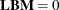(for multivariate models). Thematrix consists of one or more between-subject contrasts.

To use this feature, you must be familiar with the details of the model parameterization that PROC GLM uses. For more information, see the section Parameterization of PROC GLM Models in Chapter 46: The GLM Procedure. All the elements of thematrix can be given, or if only certain portions of thematrix are given, PROC GLMPOWER constructs the remaining elements from the context (in a manner similar to that in rule 4 in the section Construction of Least Squares Means in Chapter 46: The GLM Procedure).

There is no limit to the number of CONTRAST statements that you can specify, but they must appear after the MODEL statement. Each power analysis includes tests for all CONTRAST statements.

You can specify the following arguments:

label

identifies the contrast in the output. A label is required for every contrast that is specified. Labels must be enclosed in single or double quotation marks.

effect

identifies an effect that appears in the MODEL statement, or the INTERCEPT effect. You do not need to include all effects that appear in the MODEL statement.

values

are constants that are elements of thematrix associated with the effect.

You can specify the following option in the CONTRAST statement after a slash (/):

SINGULAR=number

tunes the estimability checking. If ABS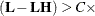number for any row in the contrast, thenis declared nonestimable.is the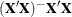matrix, and C is ABS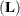except for rows whereis zero, and then it is 1. The default value for the SINGULAR= option is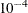. Values for the SINGULAR= option must be between 0 and 1.

As stated previously, the CONTRAST statement enables you to define custom hypothesis tests. If the hypothesis is testable in a univariate model, then the hypothesis sum of squares, SS(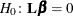), is computed as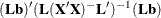where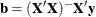.

For testable hypotheses in a multivariate model, the usual multivariate tests are defined by using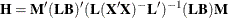where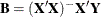and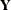is the matrix of multivariate responses or dependent variables.

The degrees of freedom associated with the hypothesis are equal to the row rank of. The sum of squares computed in this situation is equivalent to the sum of squares computed using anmatrix with any row deleted that is a linear combination of previous rows.

Multiple-degrees-of-freedom hypotheses can be specified by separating the rows of thematrix with commas.